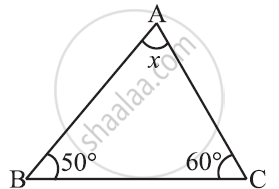Share

# Find the Value of the Unknown X In the Given Diagram: -1 - CBSE Class 7 - Mathematics

ConceptAngle Sum Property of a Triangle

#### Question

Find the value of the unknown x in the given diagram:#### Solution

The sum of all interior angles of a triangle is 180°. By using this property, these problems can be solved as follows

x + 50° + 60° = 180°

x + 110° = 180°

x = 180° − 110° = 70°

Is there an error in this question or solution?

#### APPEARS IN

NCERT Solution for Mathematics for Class 7 (2018 to Current)
Chapter 6: The Triangle and its Properties
Ex. 6.30 | Q: 1.1 | Page no. 121
Solution Find the Value of the Unknown X In the Given Diagram: -1 Concept: Angle Sum Property of a Triangle.
S